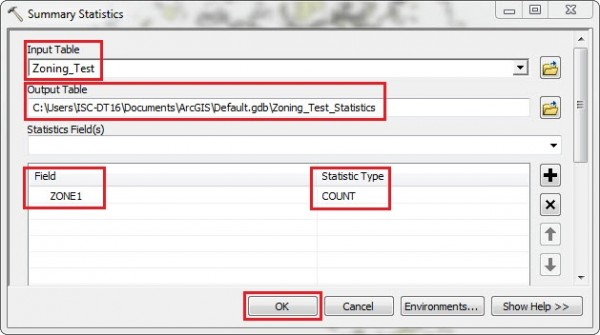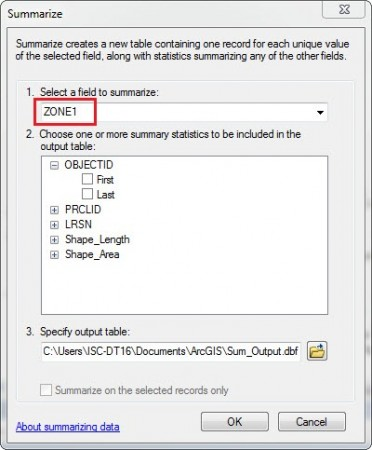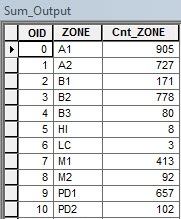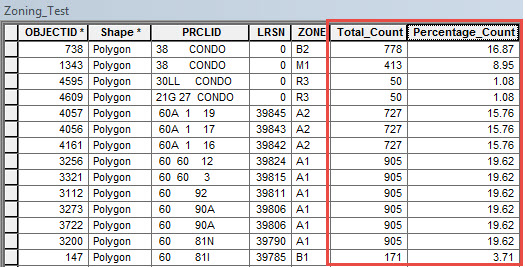English

# How To: Display the total number of parcels as percentages in the legend when using a zoning table

## Summary

When working with zoning files that display parcel data, the total amount of parcels may be expressed in terms of percentage. Displaying the parcel count in the map legend as percentage data is useful for statistical analysis and land use planning. In ArcMap, the parcel count is designed to be dynamic, which allows recalculation of the total number of parcels every time the map is updated.

To get the percentage of parcels in each zone, the values must be calculated and updated manually. The instructions provided describe how to display the total number of parcels as percentages in the legend when using a zoning table.

## Procedure

1. In ArcMap, use the Summary Statistics tool to get a total count of the parcels for every zoning type.
1. Select the zoning table as the input, and specify the location and name for the output table.
2. For Statistics Field(s), select the zone type field from the drop-down list.
3. Select COUNT from the drop-down list for Statistic Type, and click OK.1. To get the total number of parcels for each zone type, summarize the zone type field in the attribute table by right-clicking the field and selecting Summarize.
1. In the Summarize dialog box, select the field to summarize from the drop-down list.
2. Select the summary statistics to include in the output table.
3. Specify the location and the output table name, and click OK.```Note:
Click Yes when the "Do you want to add the result table in the map?" message box appears.```1. Join the summary table created in Step 2 to the zoning table using the zone type field as the common join field. To do so, right-click the zoning table, and select Joins and Relates > Join.
2. Add a new field to the zoning table to store the total number of parcels, name the field Total_Count (or any other significant name), and set the data type.
```Note:
Ensure the data type of the Total_Count field matches the data type of the parcel count field.```
1. Use the Field Calculator to populate the Total_Count field with the parcel count values from the parcel count field (joined to the table in Step 3).
2. Add another new field to the zoning table to store the percentage value of each zoning type, name the field Percentage_Count, and set the data type to Double.
3. Use the Field Calculator to populate the Percentage_Count field using the formula below:
`Percentage_Count = (Total_Count/total number of parcels) * 100`1. Symbolize the map using the percentage values, and display the values in the map legend.